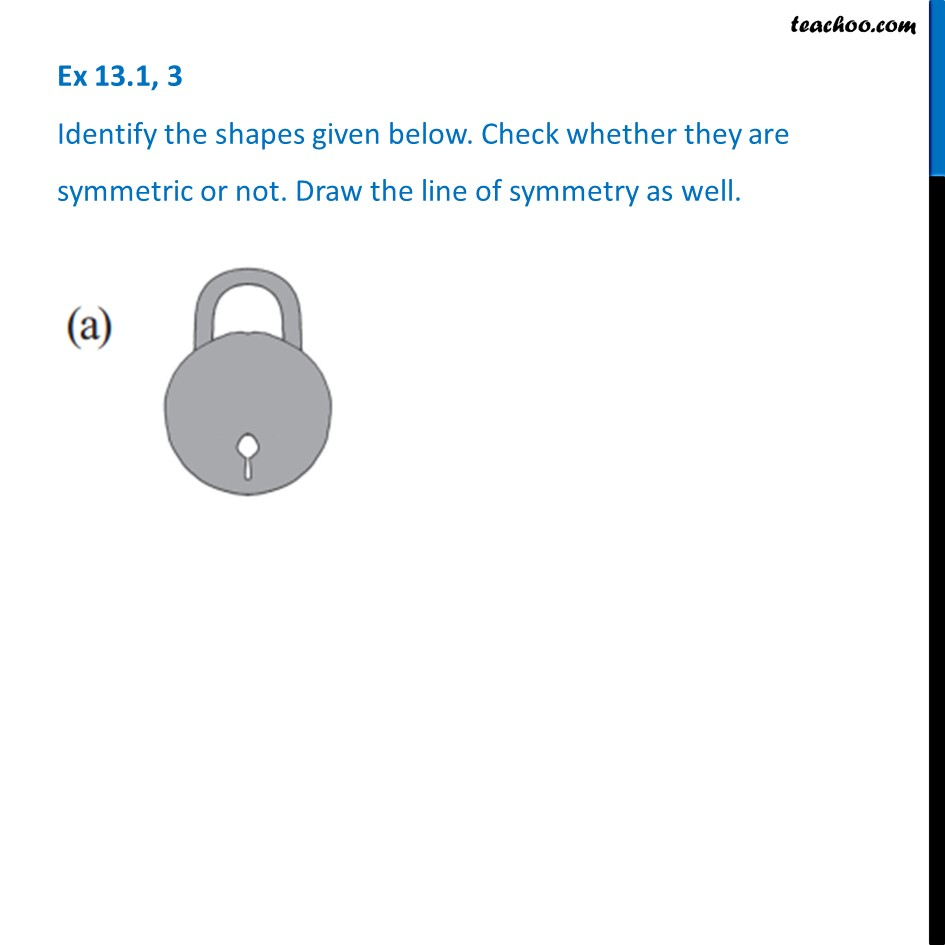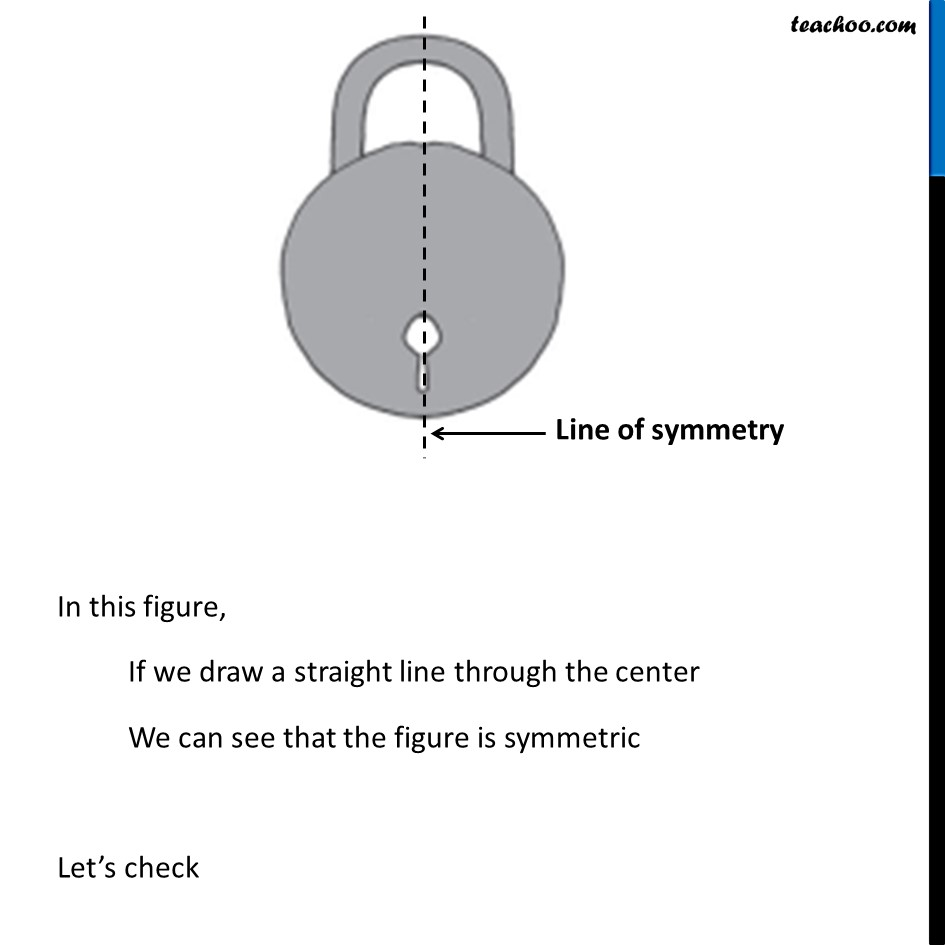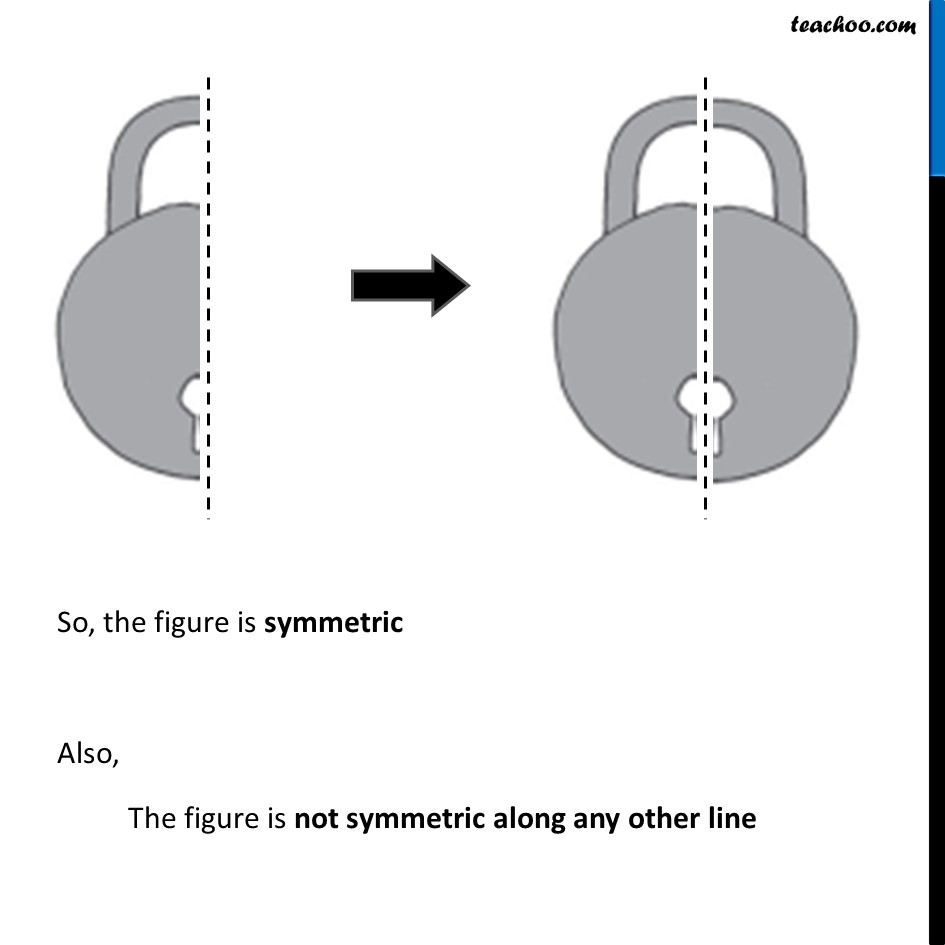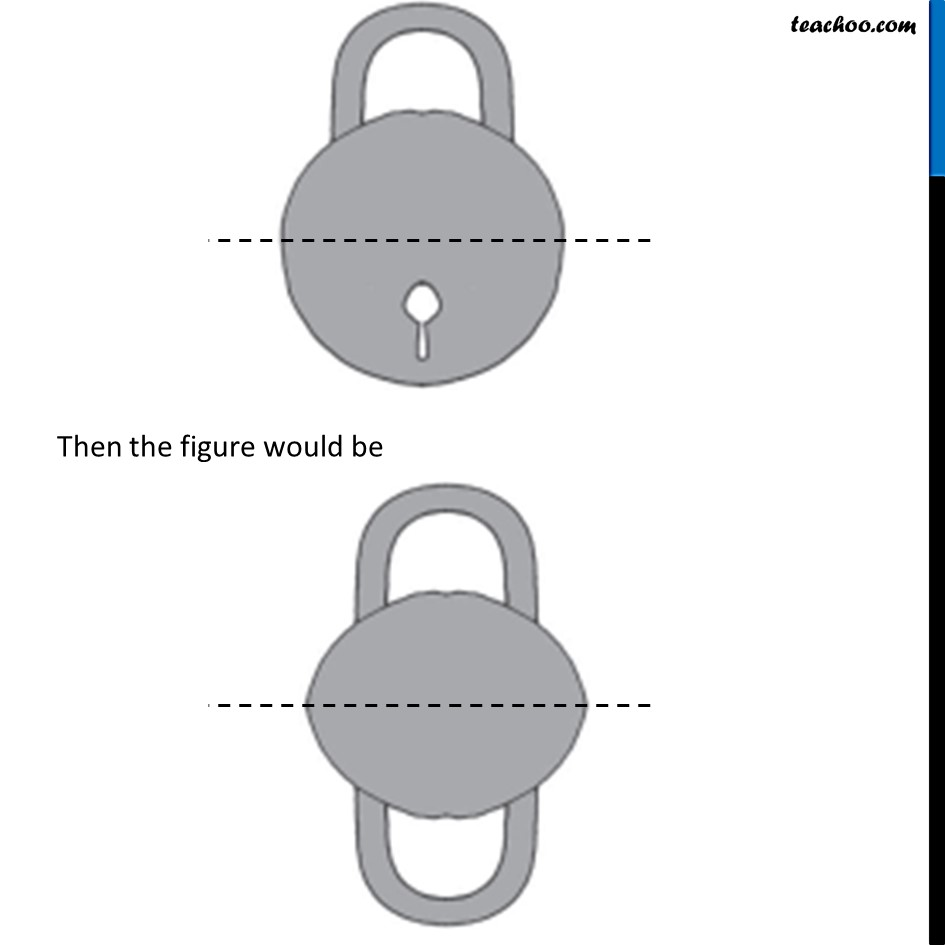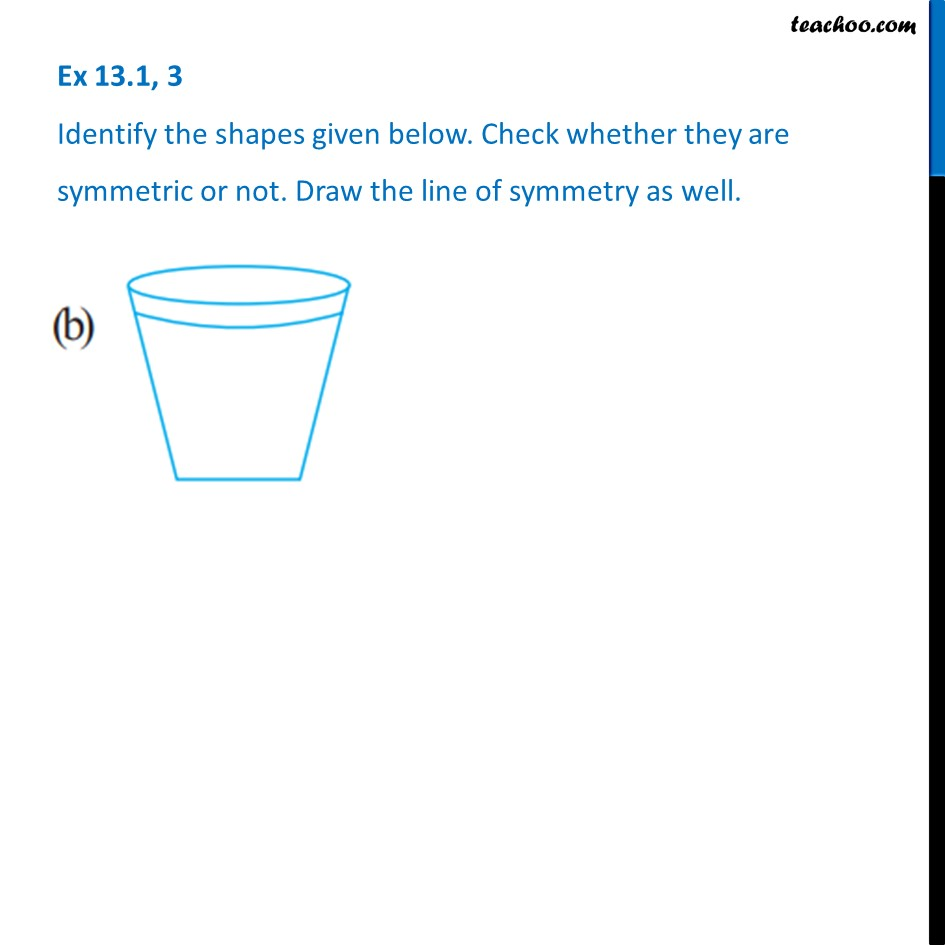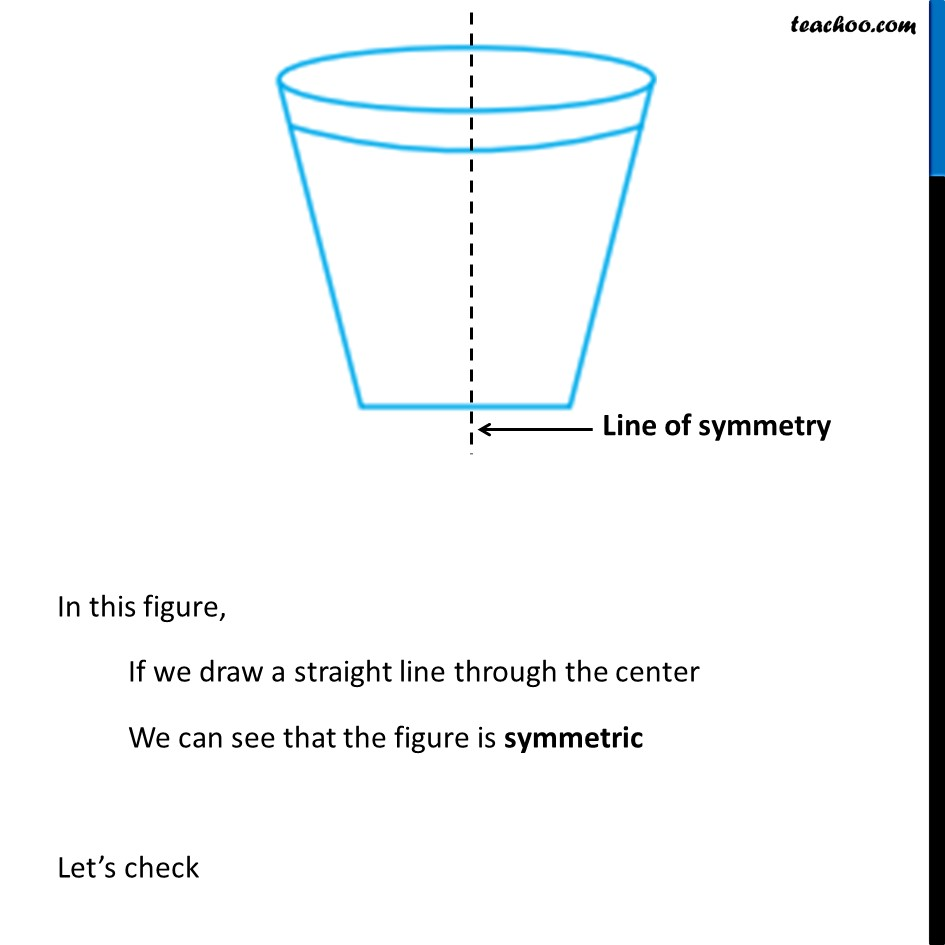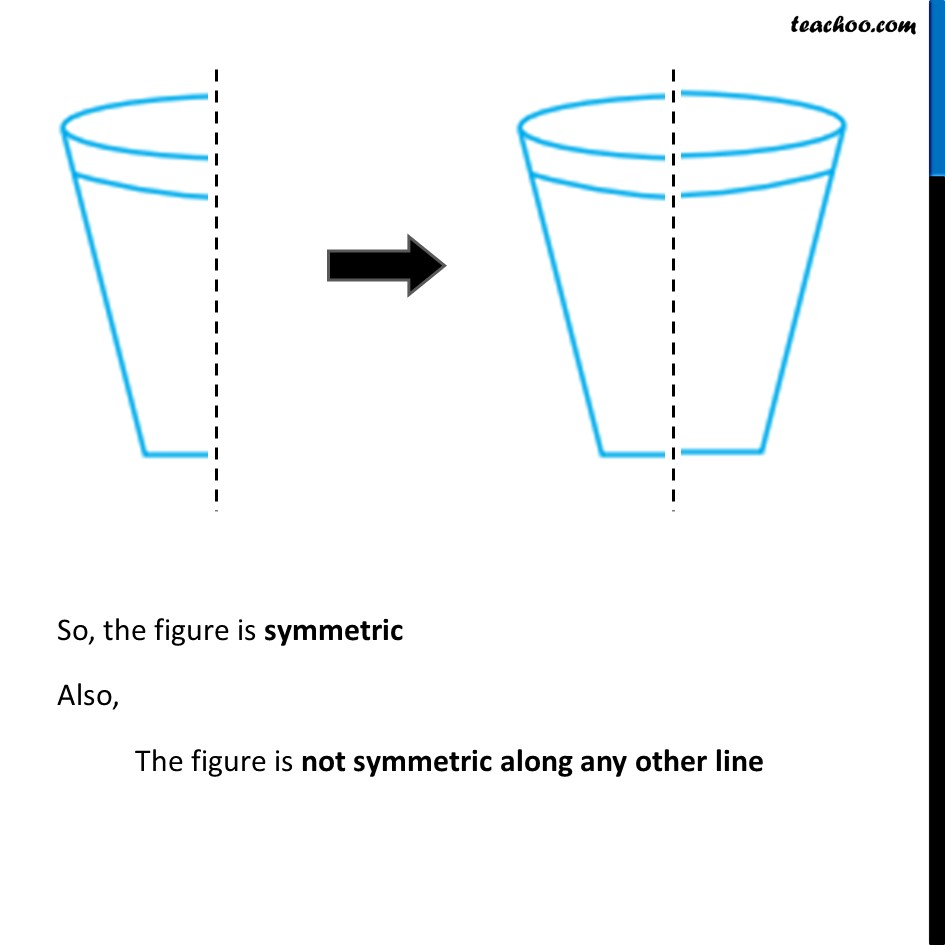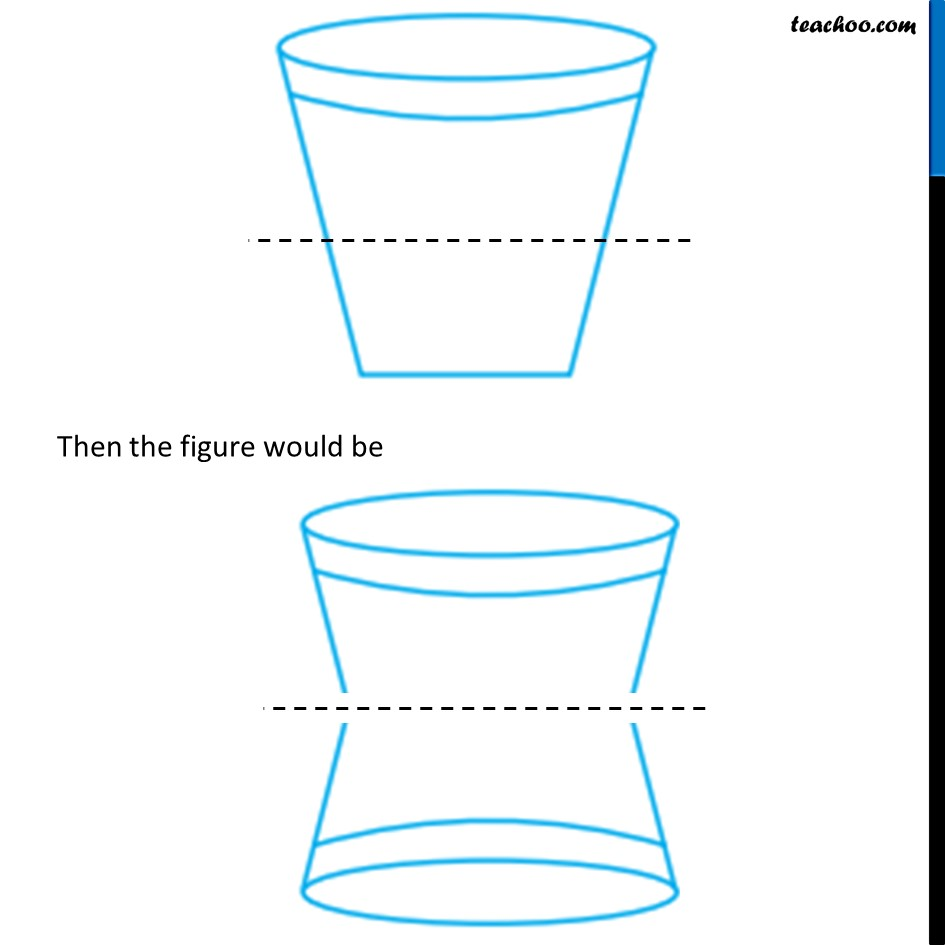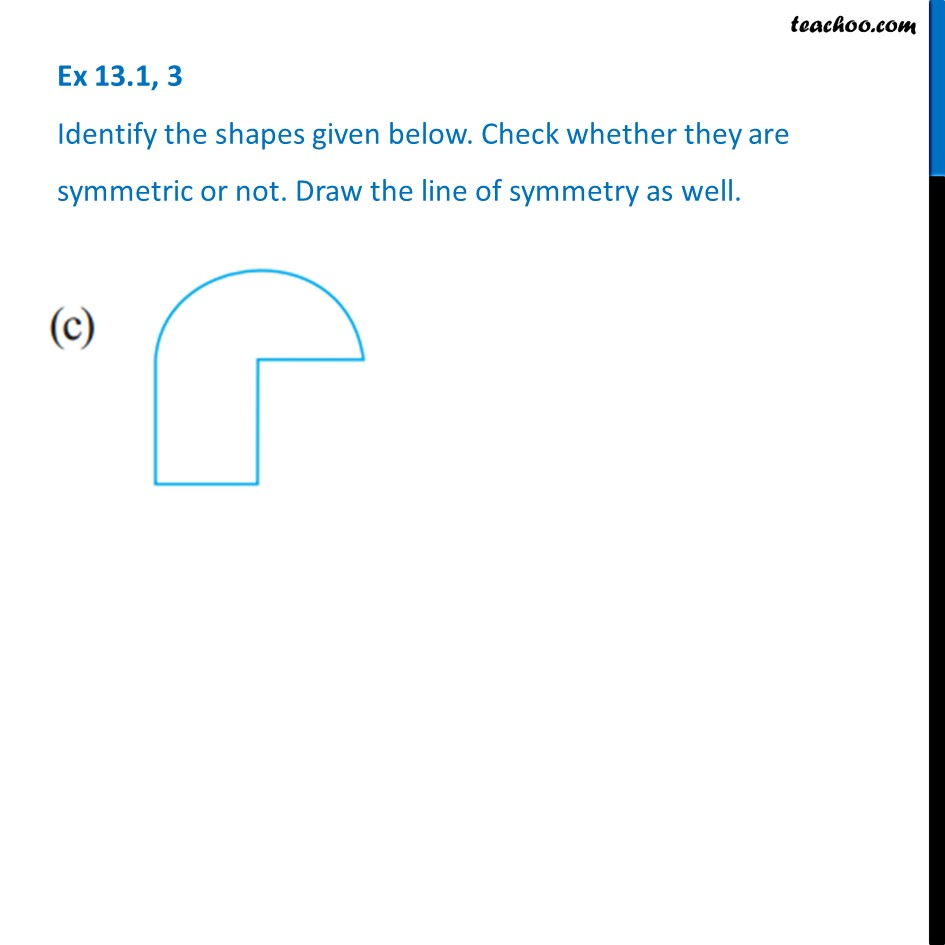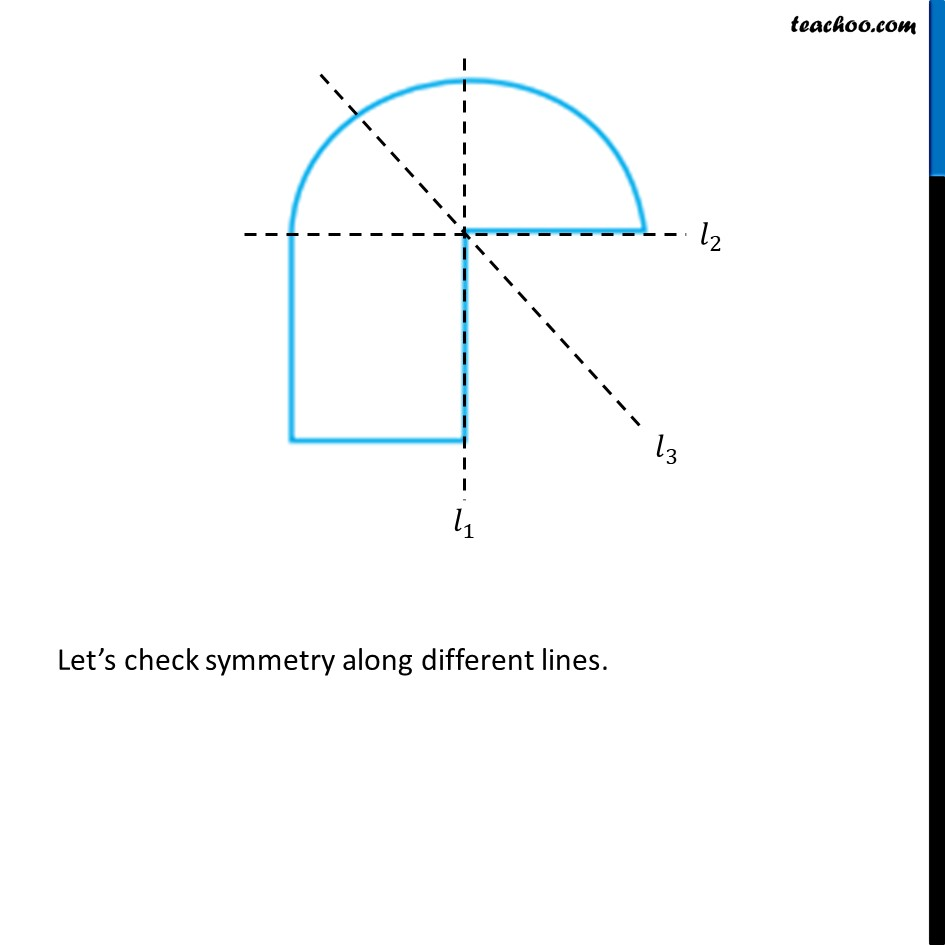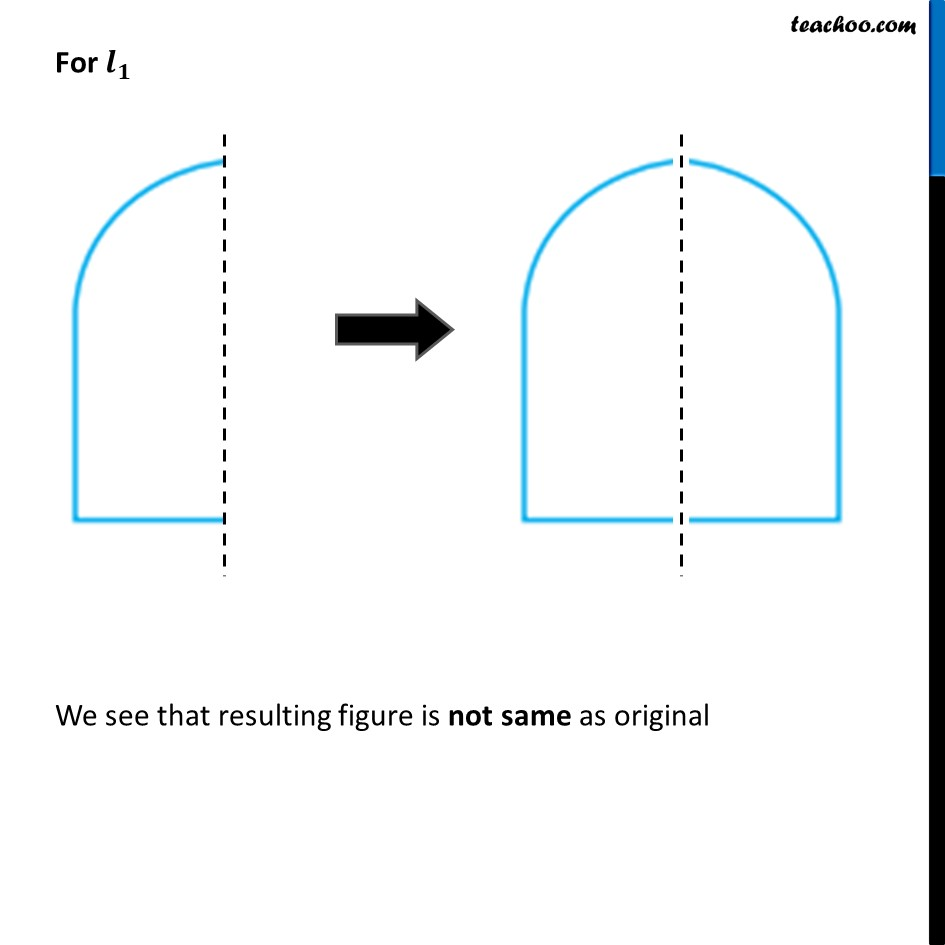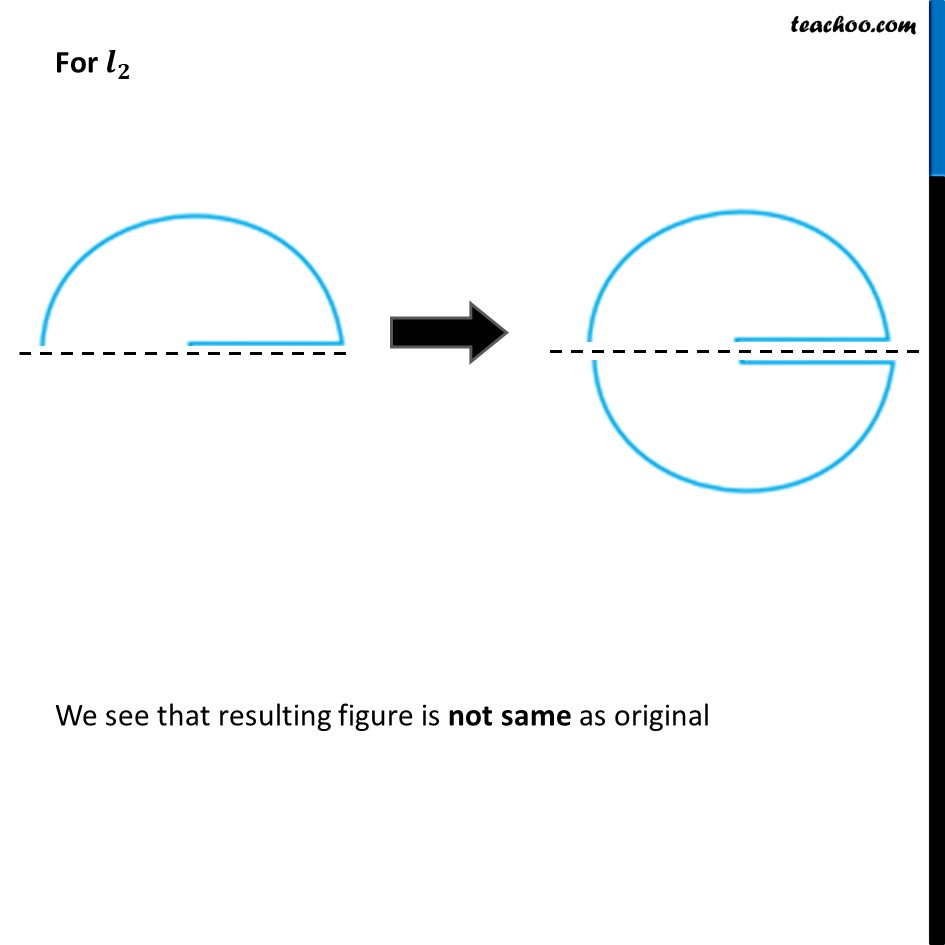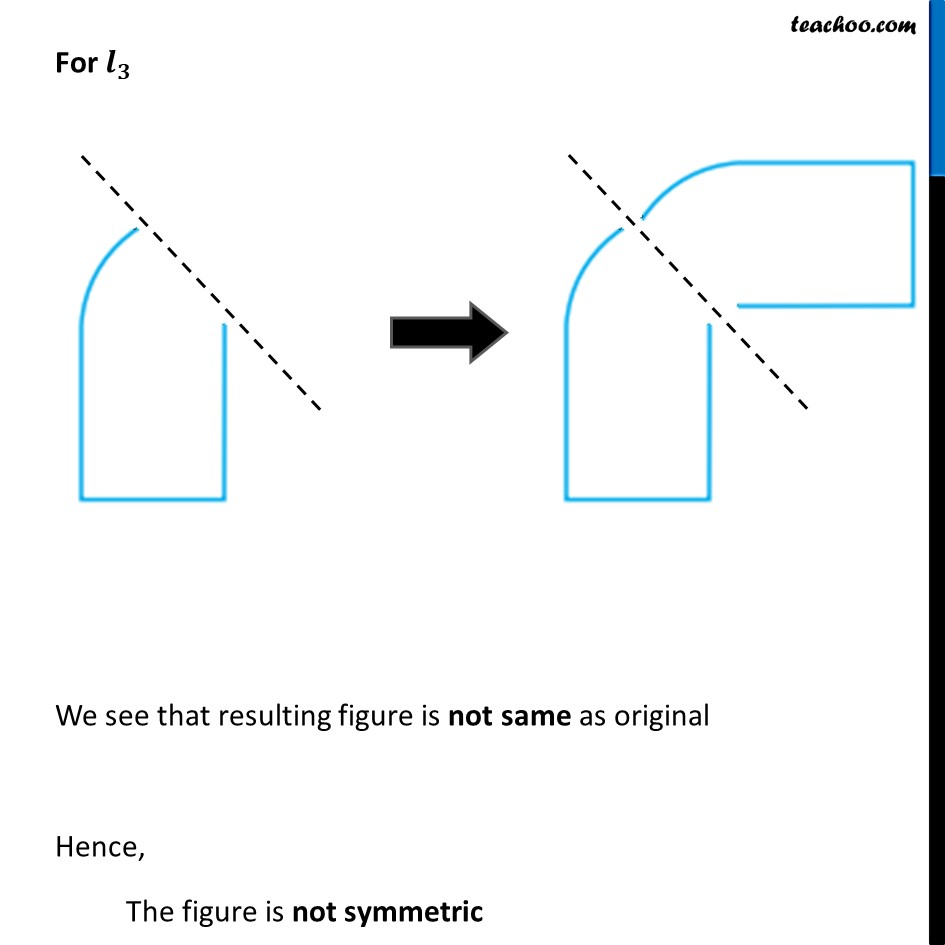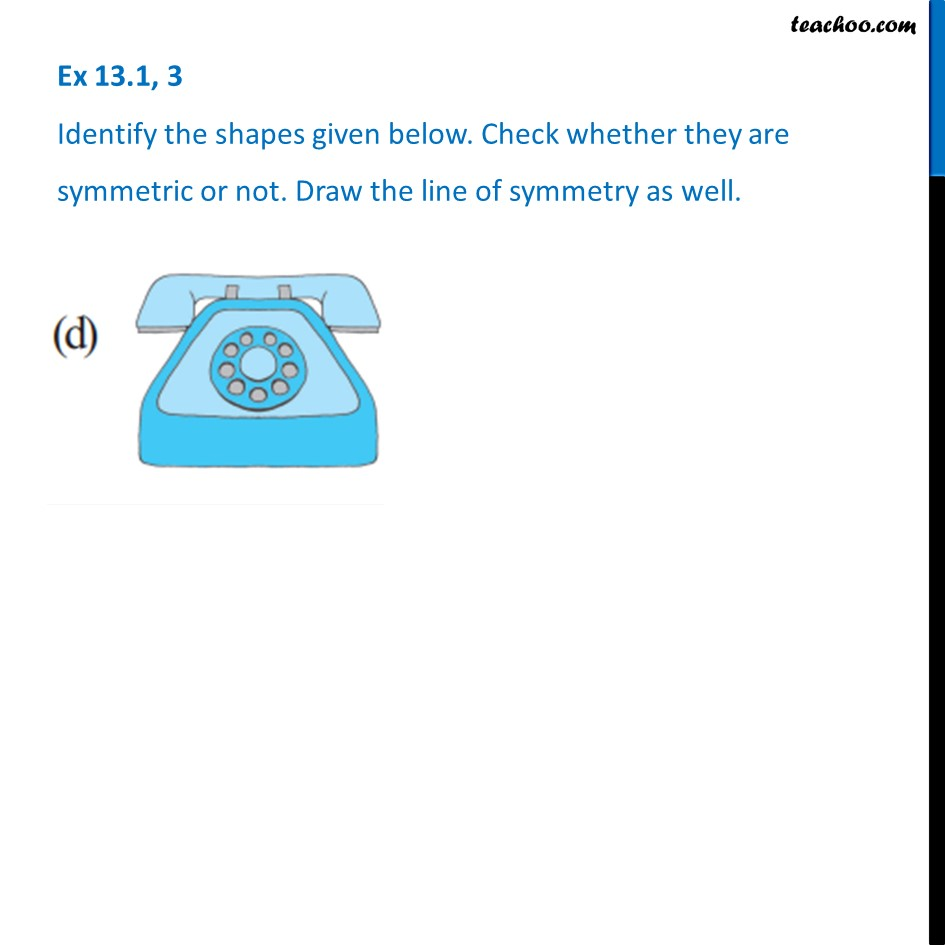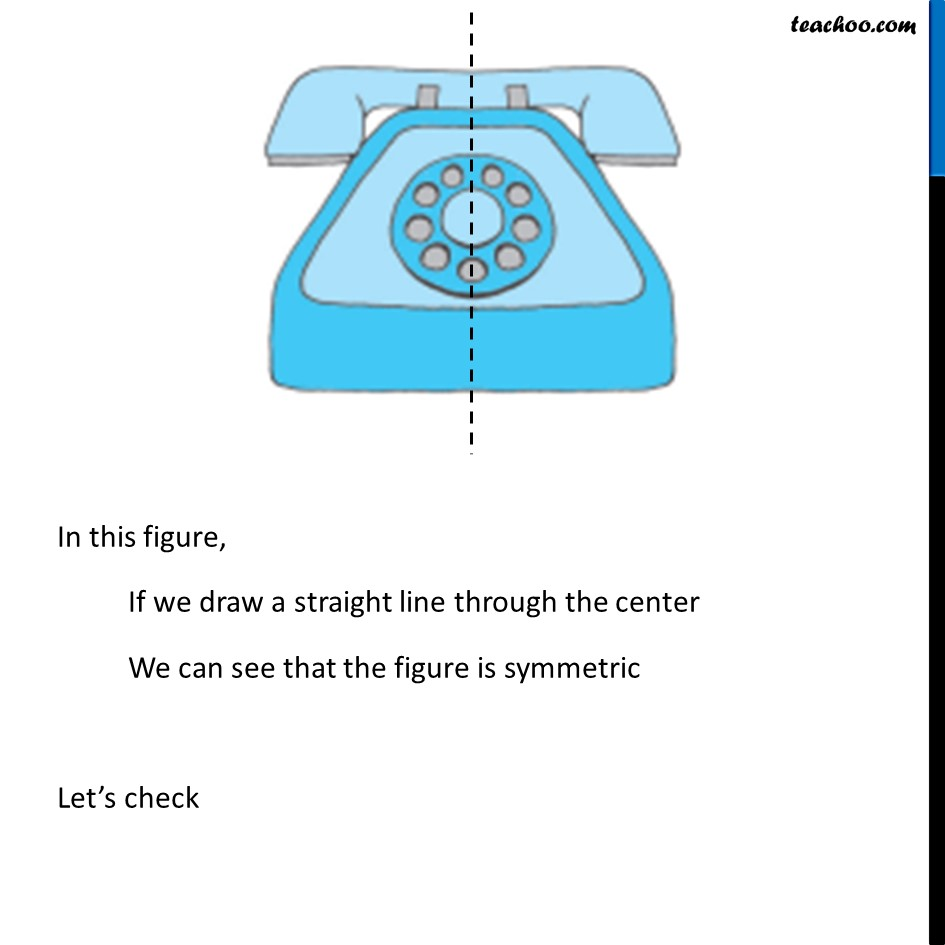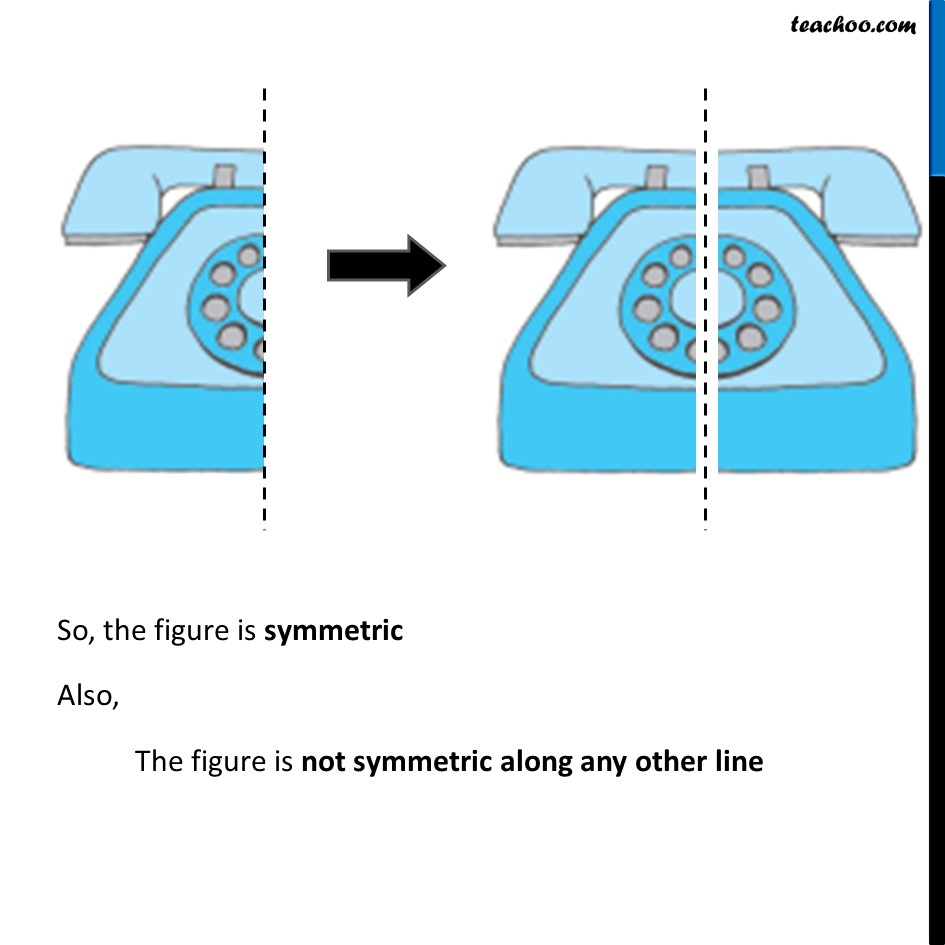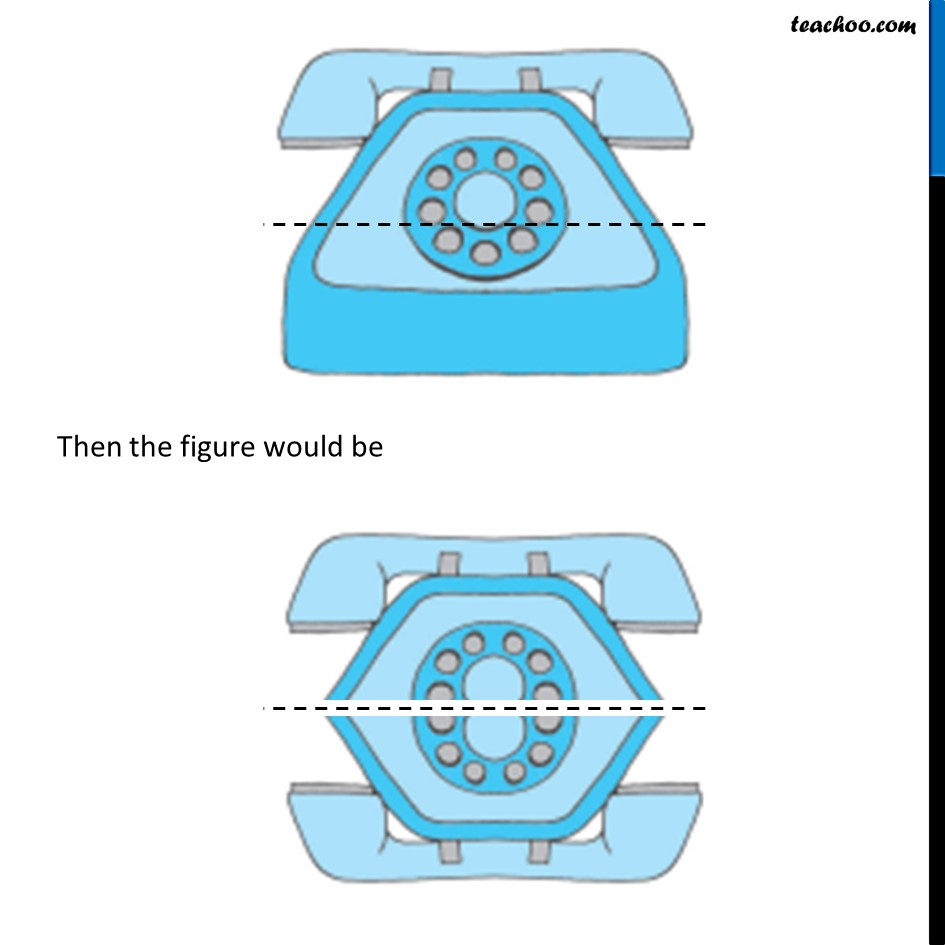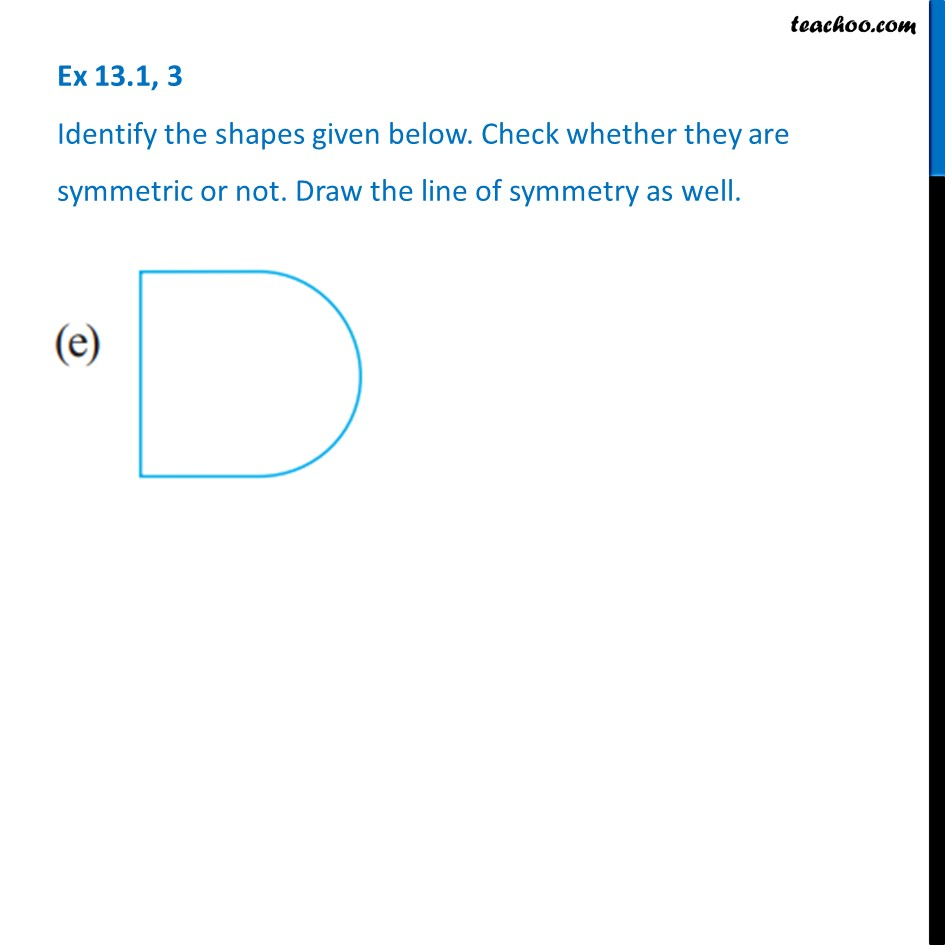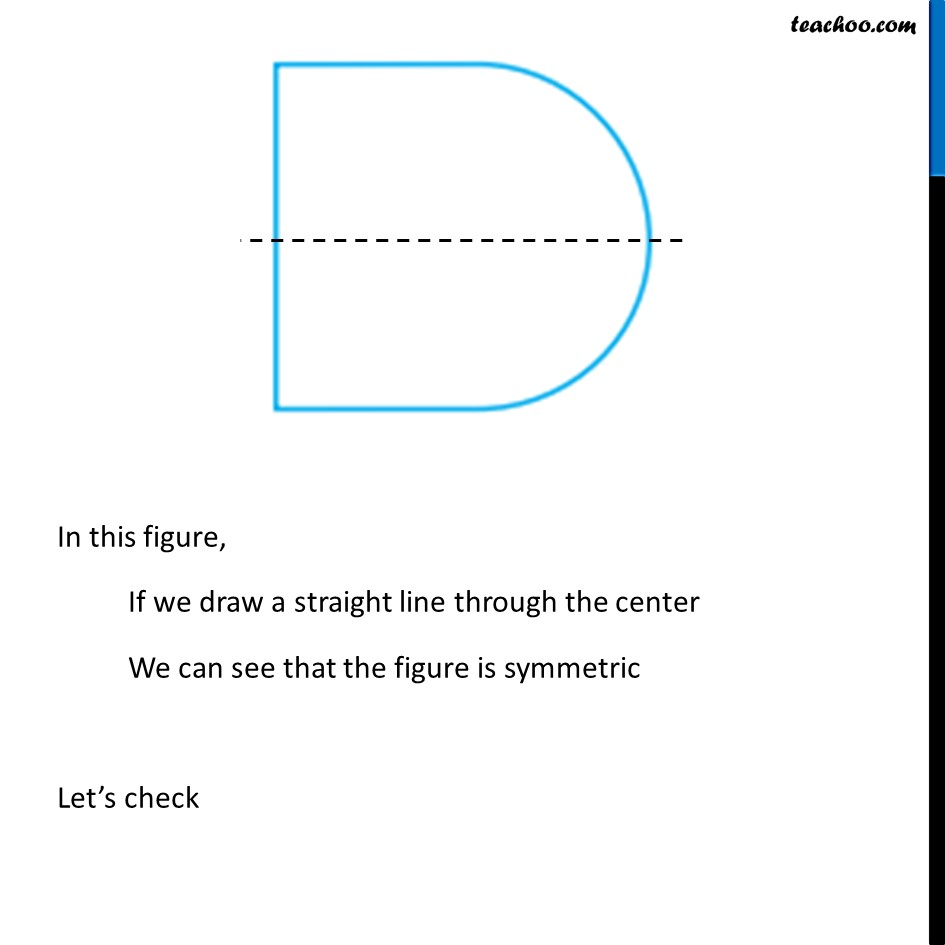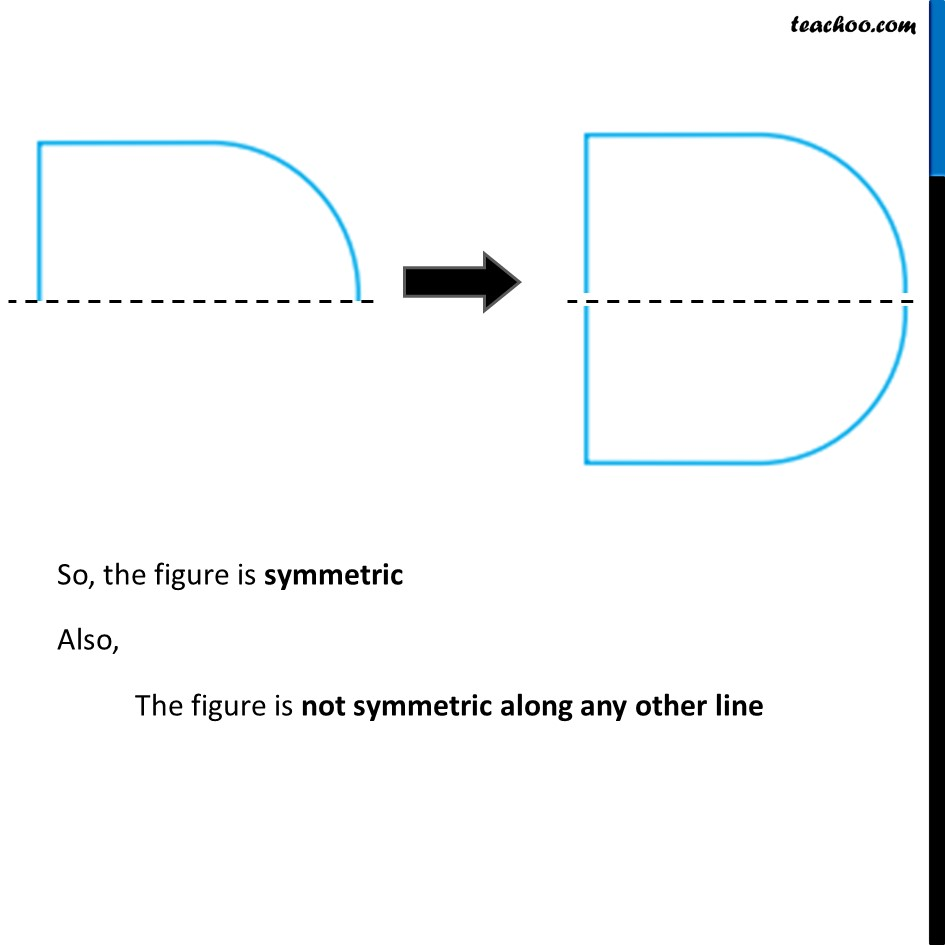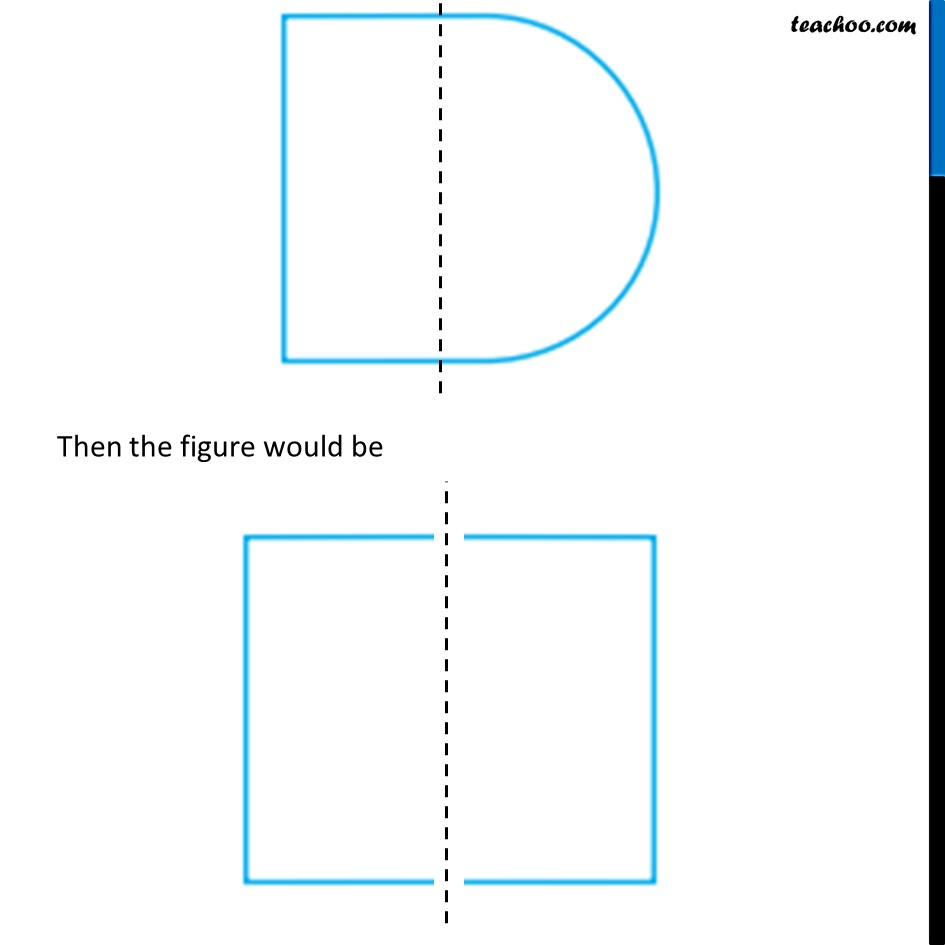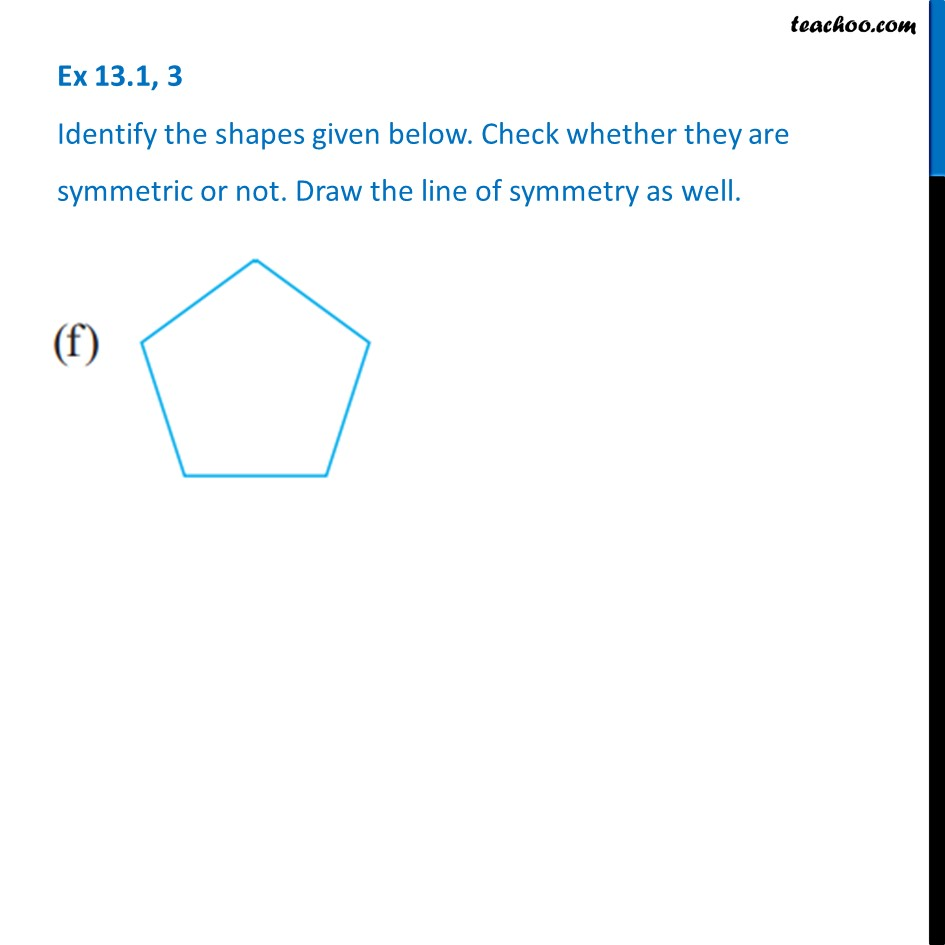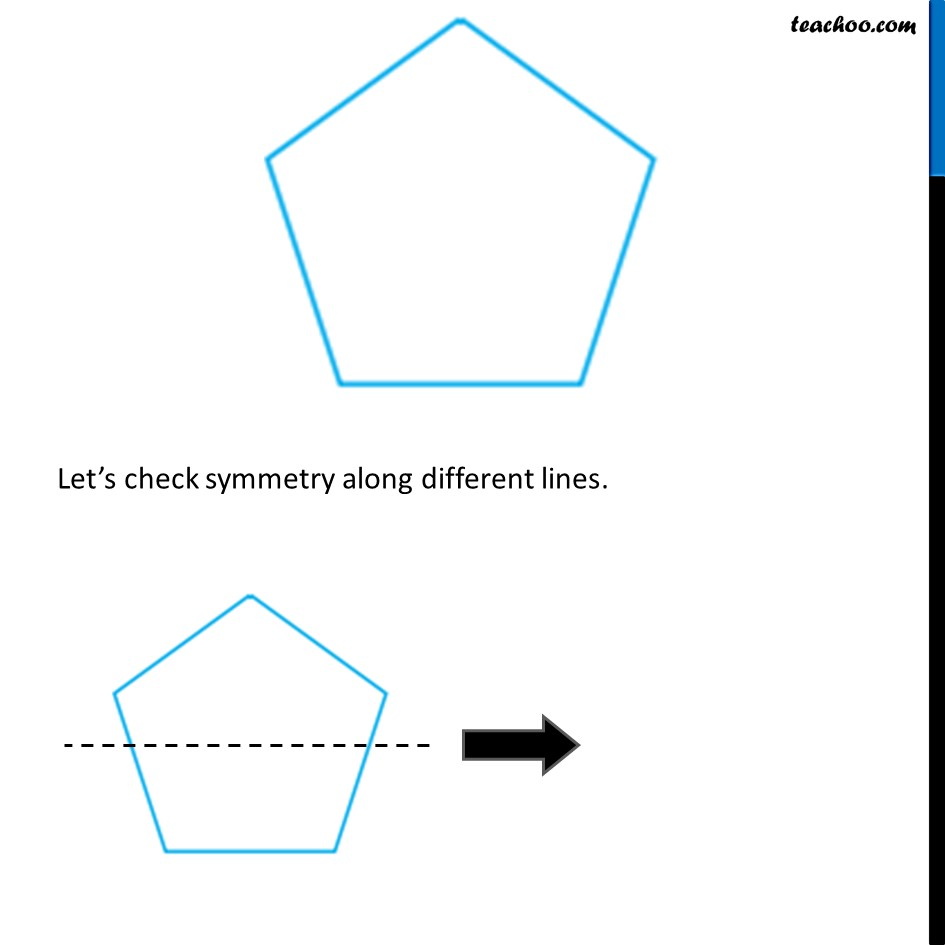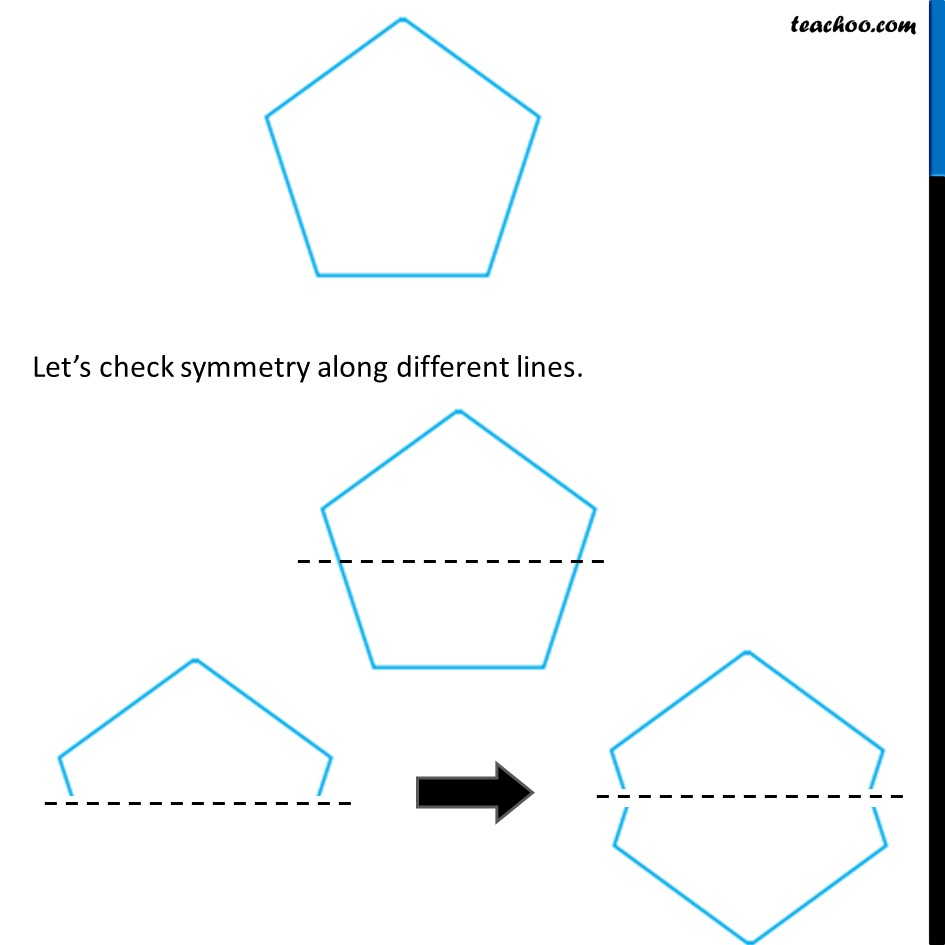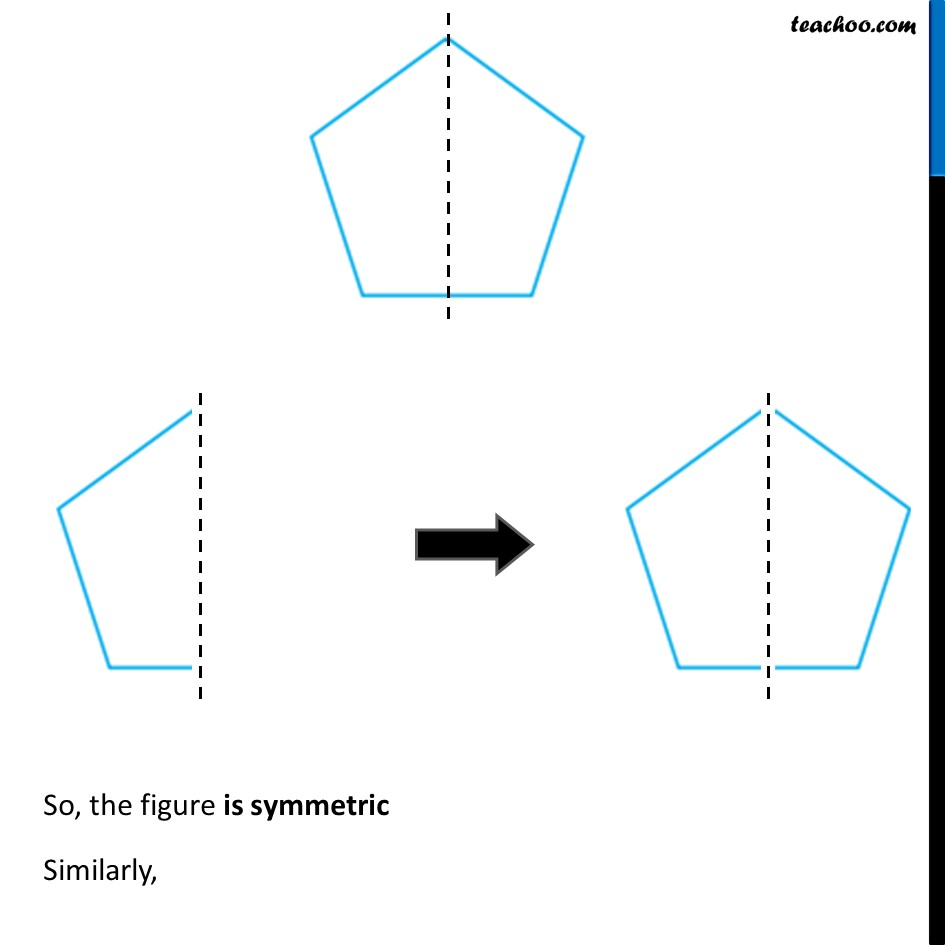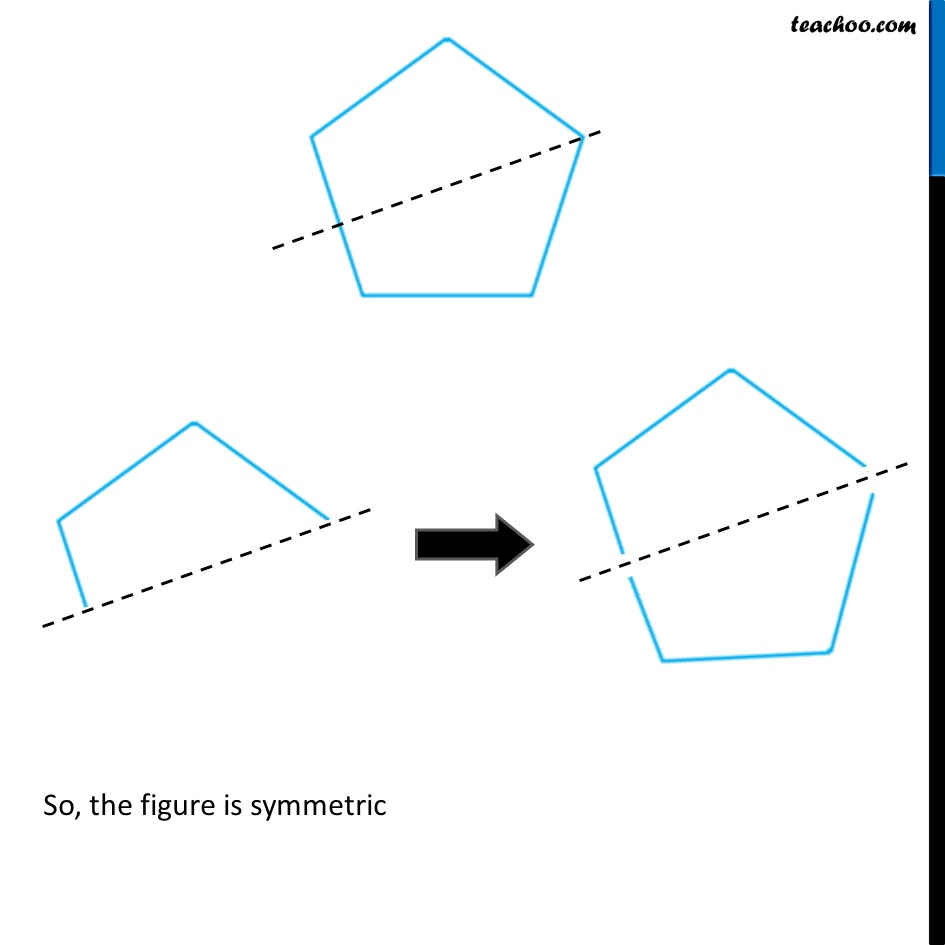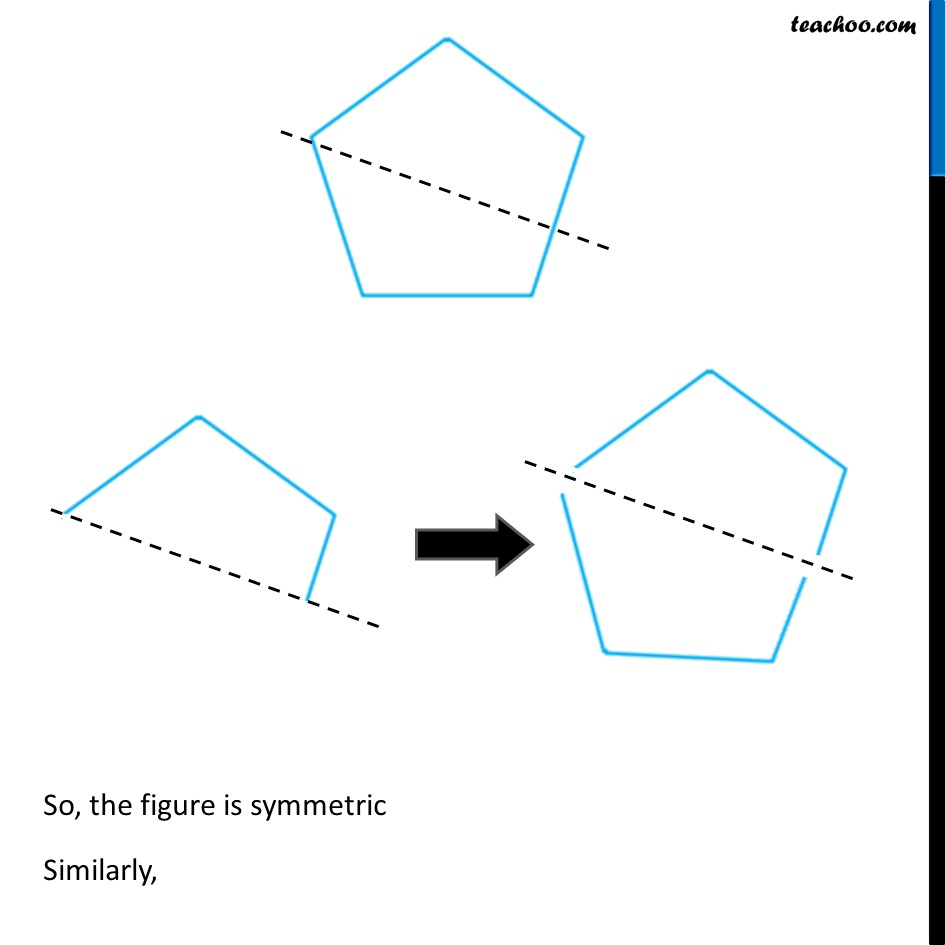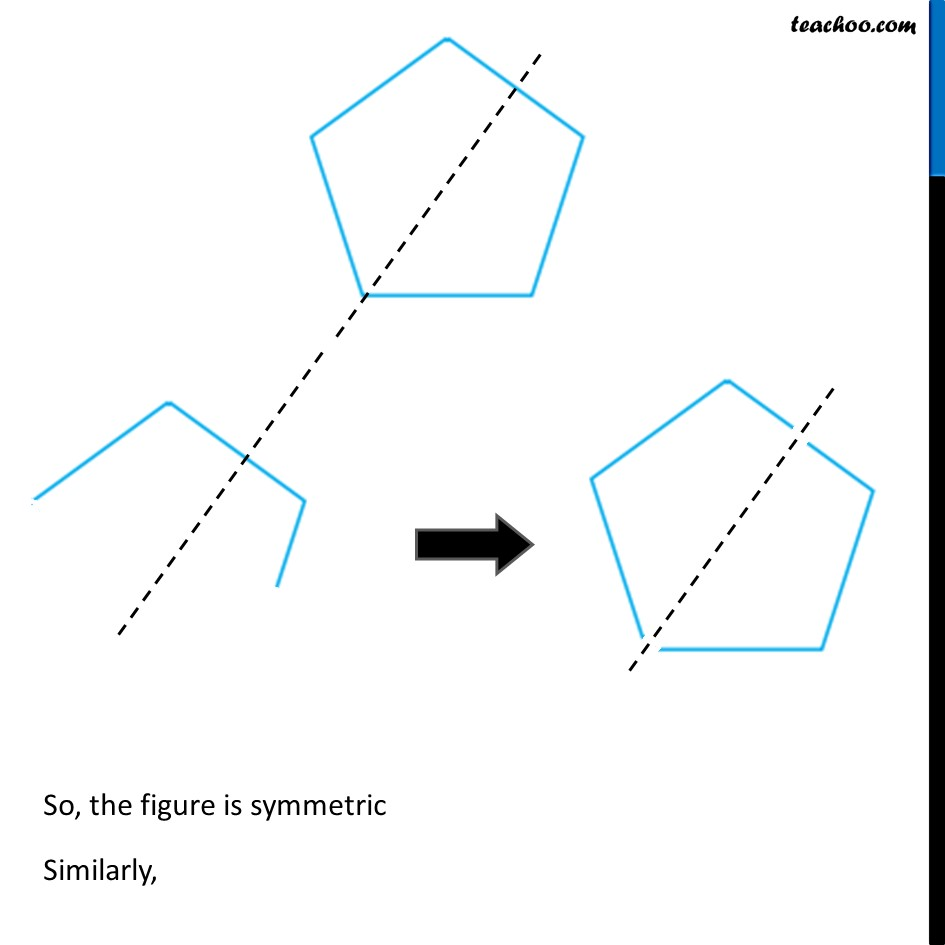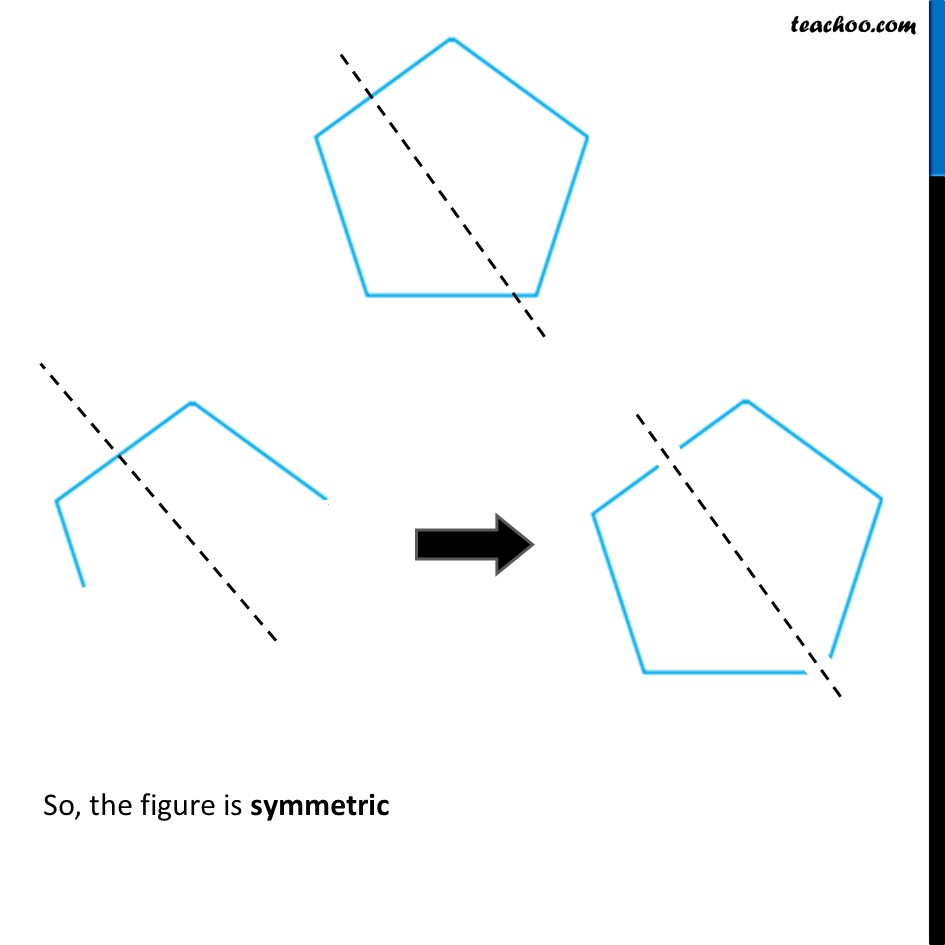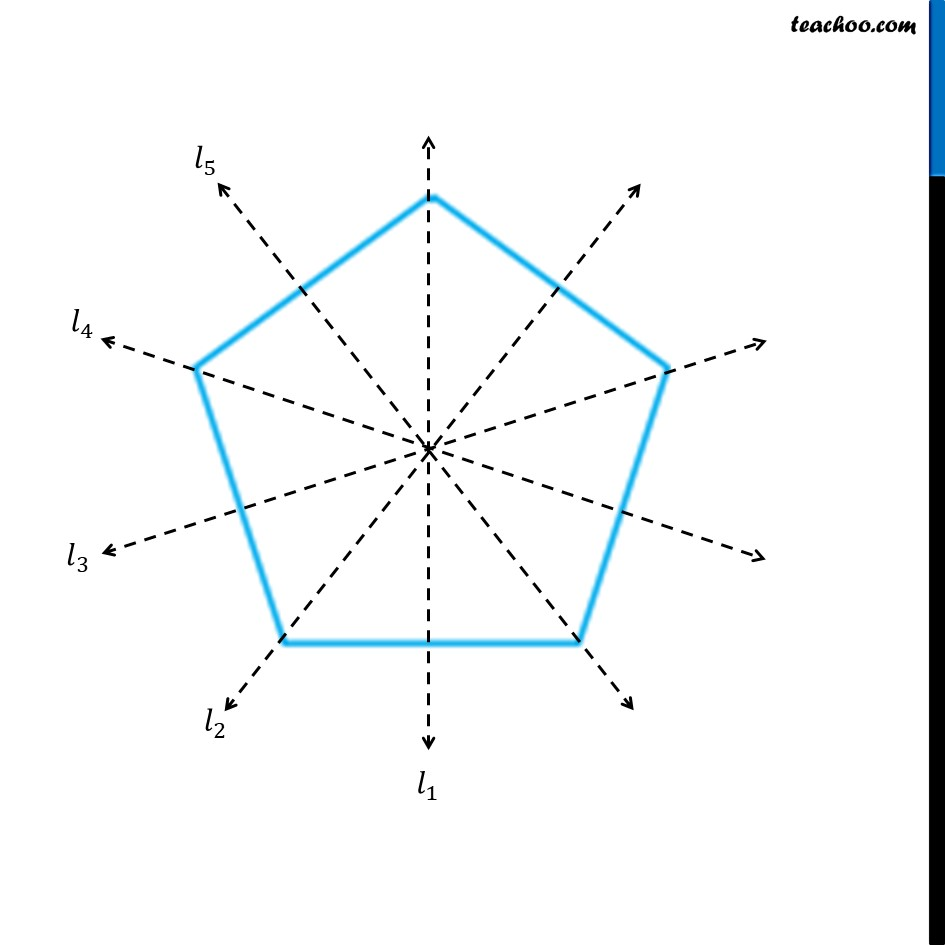1. Chapter 13 Class 6 Symmetry
2. Serial order wise
3. Ex 13.1

Transcript

Ex 13.1, 3 Identify the shapes given below. Check whether they are symmetric or not. Draw the line of symmetry as well. In this figure, If we draw a straight line through the center We can see that the figure is symmetric Let’s check So, the figure is symmetric Also, The figure is not symmetric along any other line Then the figure would be Ex 13.1, 3 Identify the shapes given below. Check whether they are symmetric or not. Draw the line of symmetry as well. In this figure, If we draw a straight line through the center We can see that the figure is symmetric Let’s check So, the figure is symmetric Also, The figure is not symmetric along any other line Then the figure would beEx 13.1, 3 Identify the shapes given below. Check whether they are symmetric or not. Draw the line of symmetry as well. In this figure, If we draw a straight line through the center We can see that the figure is symmetric Let’s check So, the figure is symmetric Also, The figure is not symmetric along any other line Then the figure would be Ex 13.1, 3 Identify the shapes given below. Check whether they are symmetric or not. Draw the line of symmetry as well.Let’s check symmetry along different lines. For 𝒍_𝟏 We see that resulting figure is not same as original For 𝒍_𝟐 We see that resulting figure is not same as original For 𝒍_𝟑 We see that resulting figure is not same as original Hence, The figure is not symmetric Ex 13.1, 3 Identify the shapes given below. Check whether they are symmetric or not. Draw the line of symmetry as well.In this figure, If we draw a straight line through the center We can see that the figure is symmetric Let’s check So, the figure is symmetric Also, The figure is not symmetric along any other line Then the figure would be Ex 13.1, 3 Identify the shapes given below. Check whether they are symmetric or not. Draw the line of symmetry as well.In this figure, If we draw a straight line through the center We can see that the figure is symmetric Let’s check So, the figure is symmetric Also, The figure is not symmetric along any other line Then the figure would be Ex 13.1, 3 Identify the shapes given below. Check whether they are symmetric or not. Draw the line of symmetry as well.Let’s check symmetry along different lines. Let’s check symmetry along different lines. So, the figure is symmetric Similarly, So, the figure is symmetric So, the figure is symmetric Similarly, So, the figure is symmetric Similarly, So, the figure is symmetric

Ex 13.1

Chapter 13 Class 6 Symmetry
Serial order wise

About the AuthorDavneet Singh
Davneet Singh is a graduate from Indian Institute of Technology, Kanpur. He has been teaching from the past 10 years. He provides courses for Maths and Science at Teachoo.